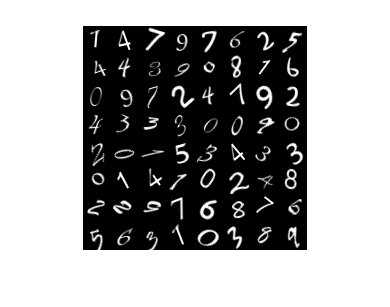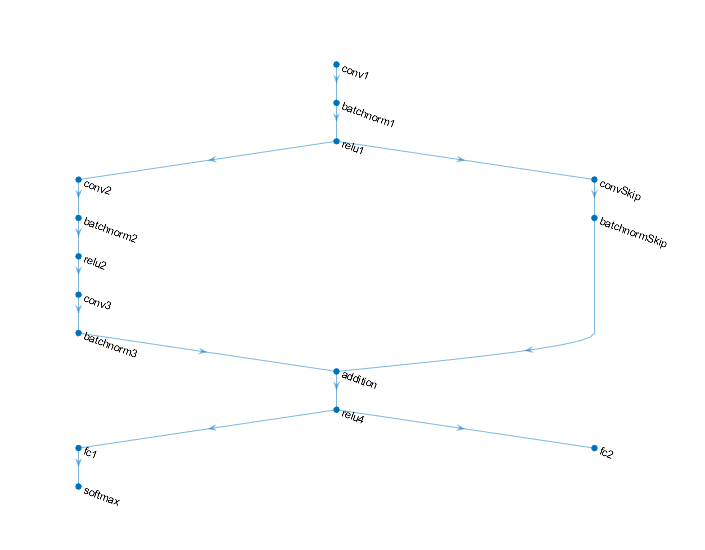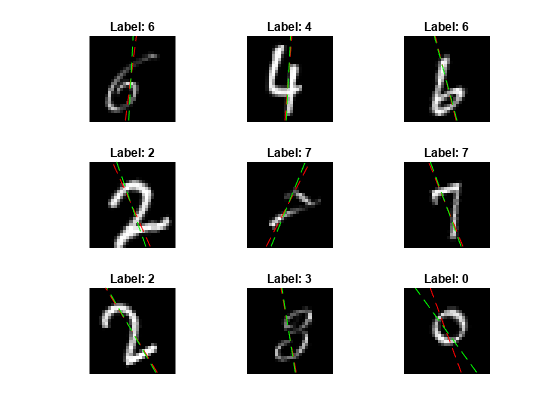# Train Network Using Model Function

This example shows how to create and train a deep learning network by using functions rather than a layer graph or a `dlnetwork`. The advantage of using functions is the flexibility to describe a wide variety of networks. The disadvantage is that you must complete more steps and prepare your data carefully. This example uses images of handwritten digits, with the dual objectives of classifying the digits and determining the angle of each digit from the vertical.

The `digitTrain4DArrayData` function loads the images, their digit labels, and their angles of rotation from the vertical. Create `arrayDatastore` objects for the images, labels, and angles, and then use the `combine` function to make a single datastore that contains all of the training data. Extract the class names and number of nondiscrete responses.

```[XTrain,T1Train,T2Train] = digitTrain4DArrayData; dsXTrain = arrayDatastore(XTrain,IterationDimension=4); dsT1Train = arrayDatastore(T1Train); dsT2Train = arrayDatastore(T2Train); dsTrain = combine(dsXTrain,dsT1Train,dsT2Train); classNames = categories(T1Train); numClasses = numel(classNames); numResponses = size(T2Train,2); numObservations = numel(T1Train);```

View some images from the training data.

```idx = randperm(numObservations,64); I = imtile(XTrain(:,:,:,idx)); figure imshow(I)```### Define Deep Learning Model

Define the following network that predicts both labels and angles of rotation.

• A convolution-batchnorm-ReLU block with 16 5-by-5 filters.

• A branch of two convolution-batchnorm blocks each with 32 3-by-3 filters with a ReLU operation between

• A skip connection with a convolution-batchnorm block with 32 1-by-1 convolutions.

• Combine both branches using addition followed by a ReLU operation

• For the regression output, a branch with a fully connected operation of size 1 (the number of responses).

• For classification output, a branch with a fully connected operation of size 10 (the number of classes) and a softmax operation.#### Define and Initialize Model Parameters and State

Define the parameters for each of the operations and include them in a struct. Use the format `parameters.OperationName.ParameterName` where `parameters` is the struct, O`perationName` is the name of the operation (for example "conv1") and `ParameterName` is the name of the parameter (for example, "Weights").

Create a structure `parameters` containing the model parameters. Initialize the learnable weights and biases using the `initializeGlorot` and `initializeZeros` example functions, respectively. Initialize the batch normalization offset and scale parameters with the `initializeZeros` and `initializeOnes` example functions, respectively.

To perform training and inference using batch normalization operations, you must also manage the network state. Before prediction, you must specify the dataset mean and variance derived from the training data. Create a structure `state` containing the state parameters. The batch normalization statistics must not be `dlarray` objects. Initialize the batch normalization trained mean and trained variance states using the `zeros` and `ones` functions, respectively.

The initialization example functions are attached to this example as supporting files.

Initialize the parameters for the first convolution operation, "conv1".

```filterSize = [5 5]; numChannels = 1; numFilters = 16; sz = [filterSize numChannels numFilters]; numOut = prod(filterSize) * numFilters; numIn = prod(filterSize) * numFilters; parameters.conv1.Weights = initializeGlorot(sz,numOut,numIn); parameters.conv1.Bias = initializeZeros([numFilters 1]);```

Initialize the parameters and state for the first batch normalization operation, "batchnorm1".

```parameters.batchnorm1.Offset = initializeZeros([numFilters 1]); parameters.batchnorm1.Scale = initializeOnes([numFilters 1]); state.batchnorm1.TrainedMean = initializeZeros([numFilters 1]); state.batchnorm1.TrainedVariance = initializeOnes([numFilters 1]);```

Initialize the parameters for the second convolution operation, "conv2".

```filterSize = [3 3]; numChannels = 16; numFilters = 32; sz = [filterSize numChannels numFilters]; numOut = prod(filterSize) * numFilters; numIn = prod(filterSize) * numFilters; parameters.conv2.Weights = initializeGlorot(sz,numOut,numIn); parameters.conv2.Bias = initializeZeros([numFilters 1]);```

Initialize the parameters and state for the second batch normalization operation, "batchnorm2".

```parameters.batchnorm2.Offset = initializeZeros([numFilters 1]); parameters.batchnorm2.Scale = initializeOnes([numFilters 1]); state.batchnorm2.TrainedMean = initializeZeros([numFilters 1]); state.batchnorm2.TrainedVariance = initializeOnes([numFilters 1]);```

Initialize the parameters for the third convolution operation, "conv3".

```filterSize = [3 3]; numChannels = 32; numFilters = 32; sz = [filterSize numChannels numFilters]; numOut = prod(filterSize) * numFilters; numIn = prod(filterSize) * numFilters; parameters.conv3.Weights = initializeGlorot(sz,numOut,numIn); parameters.conv3.Bias = initializeZeros([numFilters 1]);```

Initialize the parameters and state for the third batch normalization operation, "batchnorm3".

```parameters.batchnorm3.Offset = initializeZeros([numFilters 1]); parameters.batchnorm3.Scale = initializeOnes([numFilters 1]); state.batchnorm3.TrainedMean = initializeZeros([numFilters 1]); state.batchnorm3.TrainedVariance = initializeOnes([numFilters 1]);```

Initialize the parameters for the convolution operation in the skip connection, "convSkip".

```filterSize = [1 1]; numChannels = 16; numFilters = 32; sz = [filterSize numChannels numFilters]; numOut = prod(filterSize) * numFilters; numIn = prod(filterSize) * numFilters; parameters.convSkip.Weights = initializeGlorot(sz,numOut,numIn); parameters.convSkip.Bias = initializeZeros([numFilters 1]);```

Initialize the parameters and state for the batch normalization operation in the skip connection, "batchnormSkip".

```parameters.batchnormSkip.Offset = initializeZeros([numFilters 1]); parameters.batchnormSkip.Scale = initializeOnes([numFilters 1]); state.batchnormSkip.TrainedMean = initializeZeros([numFilters 1]); state.batchnormSkip.TrainedVariance = initializeOnes([numFilters 1]);```

Initialize the parameters for the fully connected operation corresponding to the classification output, "fc1".

```sz = [numClasses 6272]; numOut = numClasses; numIn = 6272; parameters.fc1.Weights = initializeGlorot(sz,numOut,numIn); parameters.fc1.Bias = initializeZeros([numClasses 1]);```

Initialize the parameters for the fully connected operation corresponding to the regression output, "fc2".

```sz = [numResponses 6272]; numOut = numResponses; numIn = 6272; parameters.fc2.Weights = initializeGlorot(sz,numOut,numIn); parameters.fc2.Bias = initializeZeros([numResponses 1]);```

View the structure of the parameters.

`parameters`
```parameters = struct with fields: conv1: [1×1 struct] batchnorm1: [1×1 struct] conv2: [1×1 struct] batchnorm2: [1×1 struct] conv3: [1×1 struct] batchnorm3: [1×1 struct] convSkip: [1×1 struct] batchnormSkip: [1×1 struct] fc1: [1×1 struct] fc2: [1×1 struct] ```

View the parameters for the "conv1" operation.

`parameters.conv1`
```ans = struct with fields: Weights: [5×5×1×16 dlarray] Bias: [16×1 dlarray] ```

View the structure of the state parameters.

`state`
```state = struct with fields: batchnorm1: [1×1 struct] batchnorm2: [1×1 struct] batchnorm3: [1×1 struct] batchnormSkip: [1×1 struct] ```

View the state parameters for the "batchnorm1" operation.

`state.batchnorm1`
```ans = struct with fields: TrainedMean: [16×1 dlarray] TrainedVariance: [16×1 dlarray] ```

#### Define Model Function

Create the function `model`, listed at the end of the example, that computes the outputs of the deep learning model described earlier.

The function `model` takes the model parameters `parameters`, the input data, the flag `doTraining` which specifies whether to model should return outputs for training or prediction, and the network state. The network outputs the predictions for the labels, the predictions for the angles, and the updated network state.

#### Define Model Loss Function

Create the function `modelLoss`, listed at the end of the example, that takes the model parameters, a mini-batch of input data with corresponding targets containing the labels and angles, and returns the loss, the gradients of the loss with respect to the learnable parameters, and the updated network state.

### Specify Training Options

Specify the training options. Train for 20 epochs with a mini-batch size of 128.

```numEpochs = 20; miniBatchSize = 128;```

### Train Model

Use `minibatchqueue` to process and manage the mini-batches of images. For each mini-batch:

• Use the custom mini-batch preprocessing function `preprocessMiniBatch` (defined at the end of this example) to one-hot encode the class labels.

• Format the image data with the dimension labels `"SSCB"` (spatial, spatial, channel, batch). By default, the `minibatchqueue` object converts the data to `dlarray` objects with underlying type `single`. Do not add a format to the class labels or angles.

• Train on a GPU if one is available. By default, the `minibatchqueue` object converts each output to a `gpuArray` if a GPU is available. Using a GPU requires Parallel Computing Toolbox™ and a supported GPU device. For information on supported devices, see GPU Computing Requirements (Parallel Computing Toolbox).

```mbq = minibatchqueue(dsTrain,... MiniBatchSize=miniBatchSize,... MiniBatchFcn=@preprocessMiniBatch,... MiniBatchFormat=["SSCB" "" ""]);```

For each epoch, shuffle the data and loop over mini-batches of data. At the end of each iteration, display the training progress. For each mini-batch:

• Evaluate the model loss and gradients using `dlfeval` and the `modelLoss` function.

• Update the network parameters using the `adamupdate` function.

```trailingAvg = []; trailingAvgSq = [];```

To update the progress bar of the training progress monitor, calculate the total number of training iterations.

```numIterationsPerEpoch = ceil(numObservations/miniBatchSize); numIterations = numIterationsPerEpoch * numEpochs;```

Initialize the `TrainingProgressMonitor` object. Because the timer starts when you create the monitor object, make sure that you create the object close to the training loop.

`monitor = trainingProgressMonitor(Metrics="Loss",Info="Epoch",XLabel="Iteration");`

Train the model.

```epoch = 0; iteration = 0; % Loop over epochs. while epoch < numEpochs && ~monitor.Stop epoch = epoch + 1; % Shuffle data. shuffle(mbq) % Loop over mini-batches while hasdata(mbq) && ~monitor.Stop iteration = iteration + 1; [X,T1,T2] = next(mbq); % Evaluate the model loss, gradients, and state, using dlfeval and the % modelLoss function. [loss,gradients,state] = dlfeval(@modelLoss,parameters,X,T1,T2,state); % Update the network parameters using the Adam optimizer. [parameters,trailingAvg,trailingAvgSq] = adamupdate(parameters,gradients, ... trailingAvg,trailingAvgSq,iteration); % Update the training progress monitor. recordMetrics(monitor,iteration,Loss=loss); updateInfo(monitor,Epoch=(epoch+" of "+numEpochs)); monitor.Progress = 100 * iteration/numIterations; end end```### Test Model

Test the classification accuracy of the model by comparing the predictions on a test set with the true labels and angles. Manage the test data set using a `minibatchqueue` object with the same setting as the training data.

```[XTest,T1Test,T2Test] = digitTest4DArrayData; dsXTest = arrayDatastore(XTest,IterationDimension=4); dsT1Test = arrayDatastore(T1Test); dsT2Test = arrayDatastore(T2Test); dsTest = combine(dsXTest,dsT1Test,dsT2Test); mbqTest = minibatchqueue(dsTest,... MiniBatchSize=miniBatchSize,... MiniBatchFcn=@preprocessMiniBatch,... MiniBatchFormat=["SSCB" "" ""]);```

To predict the labels and angles of the validation data, loop over the mini-batches and use the model function with the `doTraining` option set to `false`. Store the predicted classes and angles. Compare the predicted and true classes and angles and store the results.

```doTraining = false; classesPredictions = []; anglesPredictions = []; classCorr = []; angleDiff = []; % Loop over mini-batches. while hasdata(mbqTest) % Read mini-batch of data. [X,T1,T2] = next(mbqTest); % Make predictions using the predict function. [Y1,Y2] = model(parameters,X,doTraining,state); % Determine predicted classes. Y1 = onehotdecode(Y1,classNames,1); classesPredictions = [classesPredictions Y1]; % Dermine predicted angles Y2 = extractdata(Y2); anglesPredictions = [anglesPredictions Y2]; % Compare predicted and true classes Y1Test = onehotdecode(T1,classNames,1); classCorr = [classCorr Y1 == Y1Test]; % Compare predicted and true angles angleDiffBatch = Y2 - T2; angleDiff = [angleDiff extractdata(gather(angleDiffBatch))]; end```

Evaluate the classification accuracy.

`accuracy = mean(classCorr)`
```accuracy = 0.9848 ```

Evaluate the regression accuracy.

`angleRMSE = sqrt(mean(angleDiff.^2))`
```angleRMSE = single 6.5363 ```

View some of the images with their predictions. Display the predicted angles in red and the correct labels in green.

```idx = randperm(size(XTest,4),9); figure for i = 1:9 subplot(3,3,i) I = XTest(:,:,:,idx(i)); imshow(I) hold on sz = size(I,1); offset = sz/2; thetaPred = anglesPredictions(idx(i)); plot(offset*[1-tand(thetaPred) 1+tand(thetaPred)],[sz 0],"r--") thetaValidation = T2Test(idx(i)); plot(offset*[1-tand(thetaValidation) 1+tand(thetaValidation)],[sz 0],"g--") hold off label = string(classesPredictions(idx(i))); title("Label: " + label) end```### Model Function

The function `model` takes the model parameters `parameters`, the input data `X`, the flag `doTraining` which specifies whether to model should return outputs for training or prediction, and the network state `state`. The network outputs the predictions for the labels, the predictions for the angles, and the updated network state.```function [Y1,Y2,state] = model(parameters,X,doTraining,state) % Initial operations % Convolution - conv1 weights = parameters.conv1.Weights; bias = parameters.conv1.Bias; Y = dlconv(X,weights,bias,Padding="same"); % Batch normalization, ReLU - batchnorm1, relu1 offset = parameters.batchnorm1.Offset; scale = parameters.batchnorm1.Scale; trainedMean = state.batchnorm1.TrainedMean; trainedVariance = state.batchnorm1.TrainedVariance; if doTraining [Y,trainedMean,trainedVariance] = batchnorm(Y,offset,scale,trainedMean,trainedVariance); % Update state state.batchnorm1.TrainedMean = trainedMean; state.batchnorm1.TrainedVariance = trainedVariance; else Y = batchnorm(Y,offset,scale,trainedMean,trainedVariance); end Y = relu(Y); % Main branch operations % Convolution - conv2 weights = parameters.conv2.Weights; bias = parameters.conv2.Bias; YnoSkip = dlconv(Y,weights,bias,Padding="same",Stride=2); % Batch normalization, ReLU - batchnorm2, relu2 offset = parameters.batchnorm2.Offset; scale = parameters.batchnorm2.Scale; trainedMean = state.batchnorm2.TrainedMean; trainedVariance = state.batchnorm2.TrainedVariance; if doTraining [YnoSkip,trainedMean,trainedVariance] = batchnorm(YnoSkip,offset,scale,trainedMean,trainedVariance); % Update state state.batchnorm2.TrainedMean = trainedMean; state.batchnorm2.TrainedVariance = trainedVariance; else YnoSkip = batchnorm(YnoSkip,offset,scale,trainedMean,trainedVariance); end YnoSkip = relu(YnoSkip); % Convolution - conv3 weights = parameters.conv3.Weights; bias = parameters.conv3.Bias; YnoSkip = dlconv(YnoSkip,weights,bias,Padding="same"); % Batch normalization - batchnorm3 offset = parameters.batchnorm3.Offset; scale = parameters.batchnorm3.Scale; trainedMean = state.batchnorm3.TrainedMean; trainedVariance = state.batchnorm3.TrainedVariance; if doTraining [YnoSkip,trainedMean,trainedVariance] = batchnorm(YnoSkip,offset,scale,trainedMean,trainedVariance); % Update state state.batchnorm3.TrainedMean = trainedMean; state.batchnorm3.TrainedVariance = trainedVariance; else YnoSkip = batchnorm(YnoSkip,offset,scale,trainedMean,trainedVariance); end % Skip connection operations % Convolution, batch normalization (Skip connection) - convSkip, batchnormSkip weights = parameters.convSkip.Weights; bias = parameters.convSkip.Bias; YSkip = dlconv(Y,weights,bias,Stride=2); offset = parameters.batchnormSkip.Offset; scale = parameters.batchnormSkip.Scale; trainedMean = state.batchnormSkip.TrainedMean; trainedVariance = state.batchnormSkip.TrainedVariance; if doTraining [YSkip,trainedMean,trainedVariance] = batchnorm(YSkip,offset,scale,trainedMean,trainedVariance); % Update state state.batchnormSkip.TrainedMean = trainedMean; state.batchnormSkip.TrainedVariance = trainedVariance; else YSkip = batchnorm(YSkip,offset,scale,trainedMean,trainedVariance); end % Final operations % Addition, ReLU - addition, relu4 Y = YSkip + YnoSkip; Y = relu(Y); % Fully connect, softmax (labels) - fc1, softmax weights = parameters.fc1.Weights; bias = parameters.fc1.Bias; Y1 = fullyconnect(Y,weights,bias); Y1 = softmax(Y1); % Fully connect (angles) - fc2 weights = parameters.fc2.Weights; bias = parameters.fc2.Bias; Y2 = fullyconnect(Y,weights,bias); end```

### Model Loss Function

The `modelLoss` function, takes the model parameters, a mini-batch of input data `X` with corresponding targets `T1` and `T2` containing the labels and angles, respectively, and returns the loss, the gradients of the loss with respect to the learnable parameters, and the updated network state.

```function [loss,gradients,state] = modelLoss(parameters,X,T1,T2,state) doTraining = true; [Y1,Y2,state] = model(parameters,X,doTraining,state); lossLabels = crossentropy(Y1,T1); lossAngles = mse(Y2,T2); loss = lossLabels + 0.1*lossAngles; gradients = dlgradient(loss,parameters); end```

### Mini-Batch Preprocessing Function

The `preprocessMiniBatch` function preprocesses the data using the following steps:

1. Extract the image data from the incoming cell array and concatenate into a numeric array. Concatenating the image data over the fourth dimension adds a third dimension to each image, to be used as a singleton channel dimension.

2. Extract the label and angle data from the incoming cell arrays and concatenate along the second dimension into a categorical array and a numeric array, respectively.

3. One-hot encode the categorical labels into numeric arrays. Encoding into the first dimension produces an encoded array that matches the shape of the network output.

```function [X,T1,T2] = preprocessMiniBatch(dataX,dataT1,dataT2) % Extract image data from cell and concatenate X = cat(4,dataX{:}); % Extract label data from cell and concatenate T1 = cat(2,dataT1{:}); % Extract angle data from cell and concatenate T2 = cat(2,dataT2{:}); % One-hot encode labels T1 = onehotencode(T1,1); end```